# Andrej Karpathy CS294 Lecture Review: Visualizing and Understanding Deep Neural Networks

This review will go over some of the current methods that are used to visualize and understand deep neural networks.

Talk Summary: CS294 – Andrej Karpathy

I. Introduction

This review will go over some of the current methods that are used to visualize and understand deep neural networks. I will not go into too much details on the material, but rather provide some insights that I obtained while studying the material. All the original material can be found online as part of UC Berkeley’s CS294 course, presented by guest lecturer Andrej Karpathy.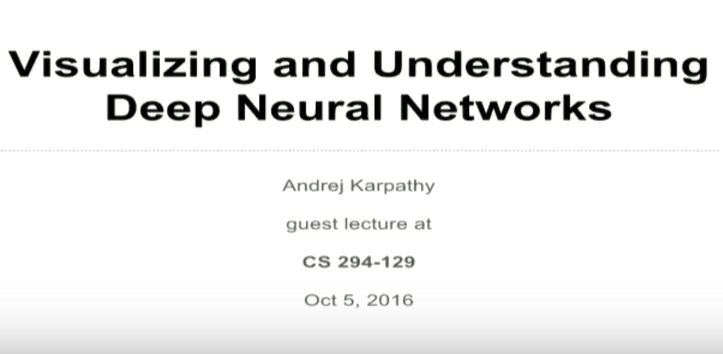II. Convex Optimization vs. Non-Convex Neural Networks

Convex optimization is a mathematically rigorous and well-studied field. Its beauty lies in its ability to derive tractable descent algorithm that arrive at global optimums. In the case of non-convex optimization problems, proof of optimality of its solutions are hard to come by. Therefore, we are concerned with our algorithms to these problems being stuck at local minima. However, this is not to say that we cannot prove optimality of non-convex problems. I’ve come across techniques that use interval analysis, provided that the function is Lipschitz continuous to some order, and its solutions (local minima) do not combinatorially explode.

Neural networks are non-convex in nature. Therefore, any formal proof of optimality have been few. The fear of being stuck at local minima was very real in the past, especially when these neural networks were first being developed (1980’s). One of the papers on this topic, The Loss Surfaces of Multilayer Net- works (by Choromanska etal. 2015), empirically shows that as the dimensions of the problem increases (think of this as having more hidden layers),the variance of the loss from your solutions decrease. Basically, the”gap” between the best and the worst solutions shrink, and all your local minima end up being about the same. Therefore, the idea of non-optimal solutions just kind of goes away. But people did not really solve this problem, it just became a non-issue.III. Layer View Representation and t-SNE (t-distributed stochastic neighbor embedding) Visualization

Simply put, Convolutional neural networks are just a giant sandwich of different layers. One way to visualize and understand these networks is to pick out a single neuron in the network and look at what “excites” this neuron? Essentially, we are empirically using activations to visualize what neurons respond to.

Another technique is to visualize the weights by training your neural networks and show the learned Gabor filters. This will only work on the very first layer of the convolutional neural network, because the weights are being convolved over the image on the first layer. As you are further down in the network, those filter results are not interpretable because their weights are convolved on top of the results from the previous layers.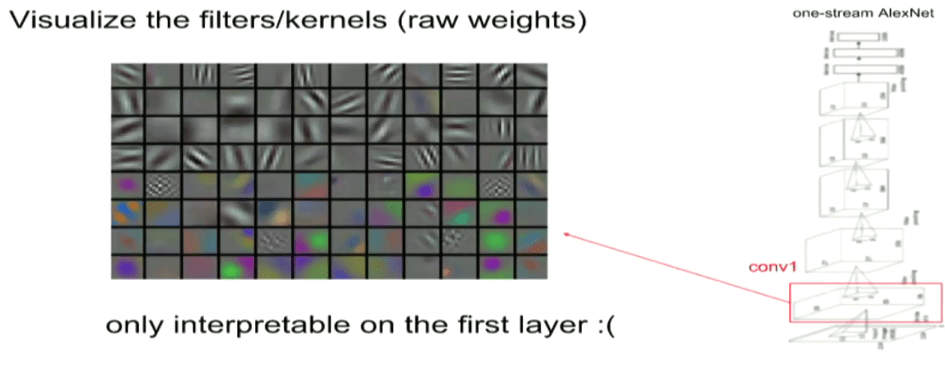Here, Andrej provides a link to the above visualization technique using ConvNetJS （https://cs.stanford.edu/people/karpathy/convnetjs/ ), it breaks down the network at each layer and you can play around with the network to inspect the gradients, activations, weights, etc. at every layer until the output class is determined.
IV. Visualizing more than just Individual Neurons

One way to look at convolutional neural networks is to look at the global representation that is achieved at the top layer as it looks at any particular image. We take an image and pass it through the network. The image is re- represented at every layer of the network, and for just one layer, we can study how the original image is embedded in that layer. To visualize this, we wish to embed these representations of the layers in a 2-D space. A nice technique to do this is the t-SNE visualization (Van der Maaten, Hinton).This technique embeds high-dimensional points into low-dimensional space such that locally pairwise distances are preserved (points that are nearby each other in low-dimensional space are also nearby each other in high-dimensional space).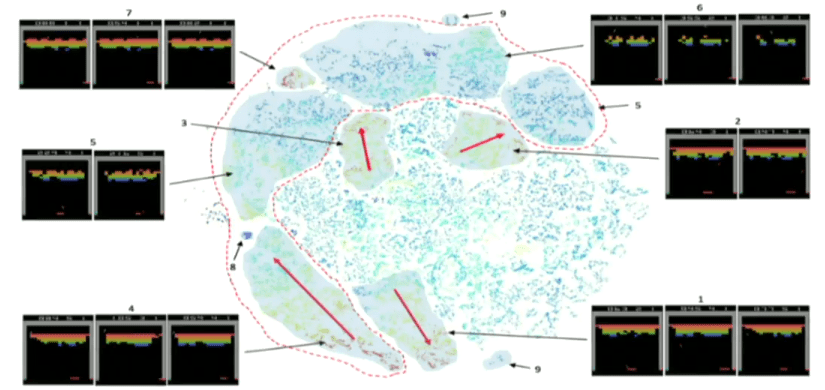V. Occlusion Experiments

This method of visualizing what the network has learned is to treat the network as a black box, then modify the input and observe the output. For example, say we have an image of a Pomeranian that is classified correctly by the network. Now we wish to “block” out certain areas of the image (by forcing the pixel values to be 0/255, or black/white) such that the output is now a function of the position of the blocked out region. We can observe that as we block out the more important features, such as the face of the Pomeranian, the probability of the network performing a correct classification drops dramatically.Here we made an interesting observation. Say we have an image of an afghan hound in the center with a man on the side of the image, and this is correctly labelled as afghan hound. If we were to cover up the man’s face with our block of 0’s, the probability of afghan hound goes up. The reason this happens is because every single image is assigned only one correct label, and when we occlude things that “compete” against the correct label, the probability of correctly labeling increases. This is also a sanity check, that the network is doing something reasonable by weighing the possibilities of what an image may be.

VI. Deconvolution Approaches

Usually we try to compute the gradient of the loss function with respect to the weights of the network, so when we do an update we can improve the weights. Let’s now consider the following question: how can we compute the gradient of any arbitrary neuron in the network with respect to the image? One way this method works is to

1. Pass an image into the network, and for some neuron that is further down in the network (neuron A)
2. Set neuron A’s gradient to one and set the gradients of all other neurons in the same layer to zero
3. Backpropagate this gradient all the way back to the image and obtain some weird looking noise-like image.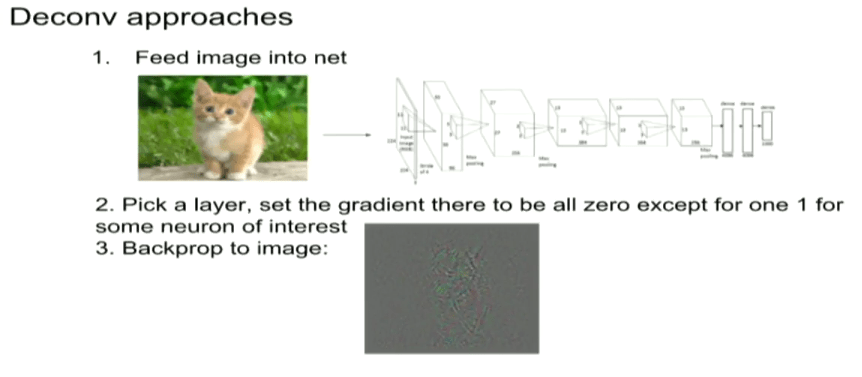This gradient image is most likely not interpretable, but what it means is that if we take this image and add it to the input image, this will increase the activation of neuron A . The backpropagation process only changes the rectifier linear unit layers. The intuition is the following: you (back) pass along the gradient to some neuron in the rectifier linear unit layer if the neuron fired, and stop the pass if the neuron did not fire.

Instead of simple backpropagation, an alternative technique to this approach is called guided backpropagation.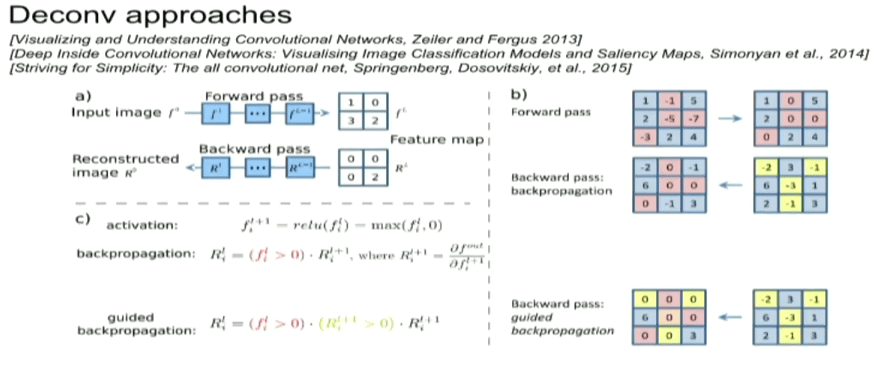This technique not only identifies whether a rectifier linear unit fired or not, but also identifies all the gradients that have negative values. Essentially, in addition to shutting off all the rectifier linear units that did not fire, all the backpropagation signals that are negative are thresholded at zero. What we end up with is passing gradients of positive contributions here. This will result in much cleaner images once backpropagated, since we have shut off all the negative influences (on the activation of the neuron that we picked) and only kept the positive influences (see Striving for Simplicity: The all Convolutional Net, Springenberg, Dosovitskiy, et al., 2015 for more information).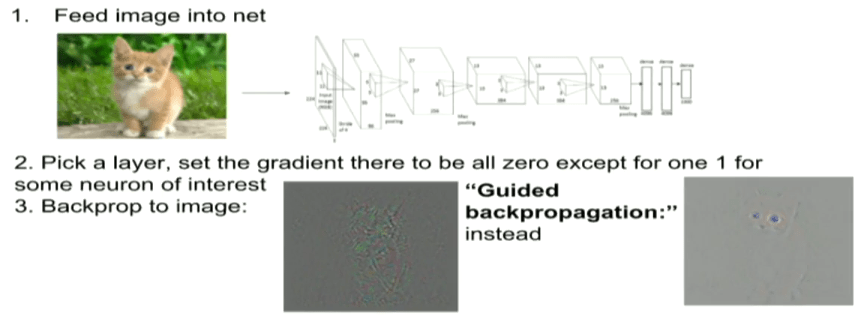VII. Optimizations to Image

i. Class VisualizationThe techniques presented next involve performing an optimization over the image.

Consider the following question: Can we find an image that maximizes some class score? To do this, we wish to keep the network fixed, use different loss functions in the network, and optimize over the image. This technique includes the following steps:

1. Feed in a random image
2. Set the gradient scores vector to be [0, 0, …, 0, 1, 0, …, 0] and backpropagate to the image
3. Do a small “image update”
4. Forward the updated image through the network
5. Repeat step 2 for some other gradient vector

By starting with a random noisy image and performing gradient ascent on some target class (backpropagating the gradient vector), we can generate an image that will improve activation of the network for that target class.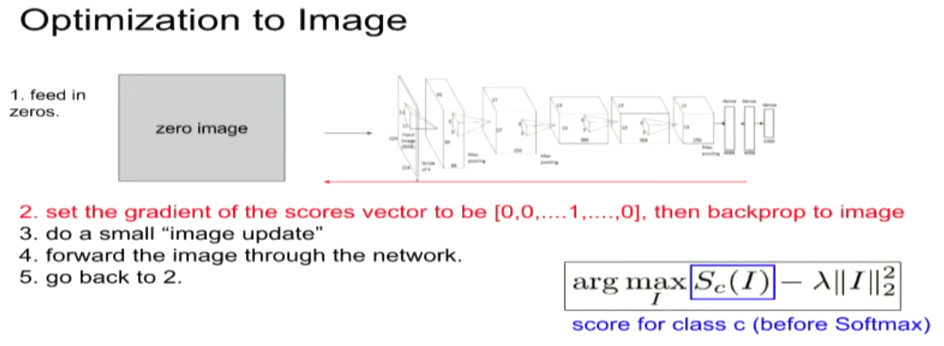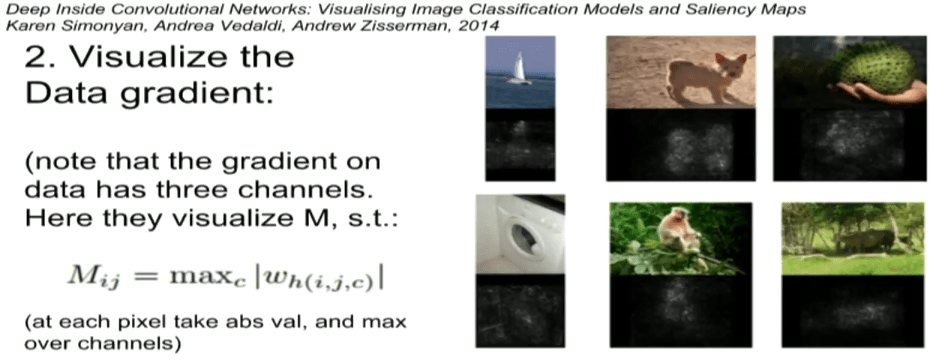Mathematically, let I be an image and let y be a target class. Let Sy ( I ) be the score that the network assigns to image I for class y. We wish to solve the following optimization problem:That is, we wish to find the image I ∗ that maximizes the score for class y assigned by the network. In the above equation, R is a regularizer term. The regularizer improves the visualization of the output image (for reference:
Simonyan, Andrea Vedaldi, and Andrew Zis- serman. “Deep Inside Convolutional Networks: Visualising Image Classification Models and Saliency Maps”, ICLR Workshop 2014). The above description was done on the very last layer – the scores of the classes.

However, the technique can be extended to any neuron in the network: start off with some random image and go forward until we reach the layer we wish to study and visualize. Then repeat the same procedure (of setting all but one gradients to zero and backpropagate to the image) for any arbitrary neuron in this layer to inspect what kind of image would maximally activate this neuron in the network. Keep in mind that regularization is included in these techniques to avoid adversarial images.

To summarize, these techniques allow us to visualize what maximizes some arbitrary scores of classes (or any arbitrary neuron along the way in the computation), subject to some regularization over the image. Different regularization schemes puts different “priors” on images of what we think is normal image, therefore it will influence the outcome of these experiments drastically.

ii. Feature Inversion

Another question we could ask is: Given a convolutional neural network code (think of this as the output at some layer in the network), is it possible to reconstruct the original image? The following technique tries to reconstruct an image from some given code (feature representation). The steps to this experiment are as follows:

1. Feed some input image through the network
2. Discard the input image
3. We backpropagate the outputs at some layer further down in the network to generate images that have identical code (feature representation learned at this layer)

Mathematically, let I be an input image, and φl ( I ) be the activations at layer l of the convolutional network φ. We wish the solve the following optimization problem: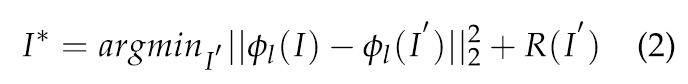That is, we wish to find the image I ∗ that maximizes the score for class y assigned by the network. In the above equation, R is a regularizer term. The regularizer improves the visualization of the output image (for explicit regularizers and their effects, see Karen Simonyan, Andrea Vedaldi, and Andrew Zisserman. “Deep Inside Convolutional Networks: Visualising Image Classification Models and Saliency Maps”, ICLR Workshop 2014). The above description was done on the very last layer, on the scores of the classes. However, the technique can be extended to any neuron in the network. We start off with some random image and forward until the layer we wish to study and visualize. We repeat the same procedure (of setting all but one gradients to zero and backpropagate to the image) for any arbitrary neuron in this layer to inspect what kind of image would maximally activate this neuron in the network. Keep in mind that regularization is included in these techniques to avoid adversarial images.

To summarize, these techniques allow us to visualize what maximizes some arbitrary scores of classes (or any arbitrary neuron along the way in the computation), subject to some regularization over the image. Different regularization schemes puts different “priors” on images of what we think is normal image, so will influence the outcome of these experiments drastically.

VIII. Adversarial Images for ConvNets

An adversarial image is an image formed by adding small but intentionally worst-case perturbations to images from the dataset, such that the network will classify the newly formed image incorrectly with high probability. In practice, we can take any image that has the correct label when fed through the network, and perturb it with some adversarial image such that the differences between the two images are imperceptible, but the end result will be classified incorrectly.

Without going into too much mathematical details, the reason why this happens is due to the linear nature within neural networks which almost always have high dimensions. Intuitively, consider the following linear example: let x be an input image and w as the weights of some model. The computations to obtain the output is defined by the inner product between x and w: wTx. If we perturb the input slightly by η to obtain x¯ = x + η, the output becomes wT x¯ = wT x + wT η. The adversarial perturbation causes the activation to grow by wTη. We can therefore maximize this term to be subjected to some constraints (typically norm constraints) on η to cause problems in the model. However, as the dimensions of the problem grow, we can make many small perturbations to the vector η while satisfying the norm constraint, and all these infinitesimal changes eventually add up to one large change to the output. This is just a (extremely) simple intuition of how I interpret the effect of linearity has on the results of adversarial images

Another way to look at these adversarial images across different models is to think of them as a result of the adversarial perturbations being highly aligned with the weight vectors of a model. This is due to the explanation above, where those infinitesimal changes force the linear model to attend exclusively to the signal that most closely aligns with its weights, even if the other signals(from the correct image) may have greater amplitude.IX. Deep Dream Experiments

The intuition behind deep dream experiments is quite simple. We are essentially modifying the image to amplify the activations at some chosen layer in the network. To do this, we:

1. Pick some layer from the network
2. Pass some input image through the network to extract features at the chosen layer
3. Set the gradient at that layer to the activations themselves
4. Backpropagate to the image.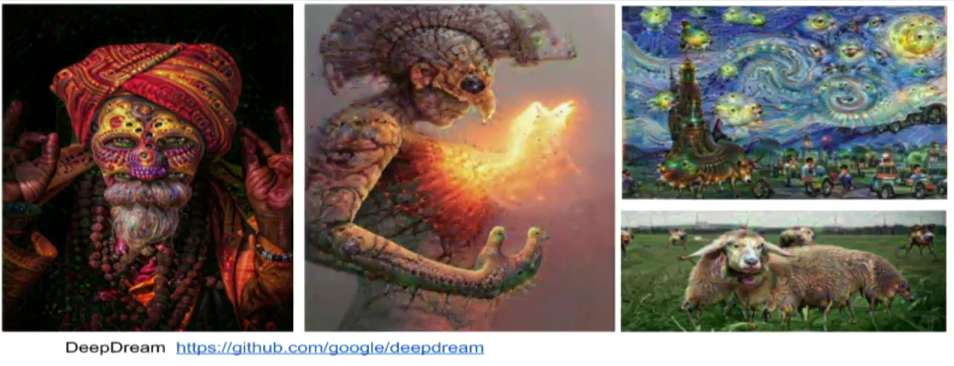X. Neural Style Experiments

Consider the following setup, we have two images: one content image Ic and one style image Is . We would like to generate a third image that has the content of Ic and the style of Is . In other words, what we wish to do is extract the content from Ic and extract the style of Is .To extract the content, we are going to pass Ic through our neural network and simply store the activations at each layer.

To extract the style is a bit different. We pass Is through our network, and compute the Gramian matrix (G = V T V) at every single layer of these activations. From a algebraic point of view, the Gramian matrix G is just all the inner products of columns of V.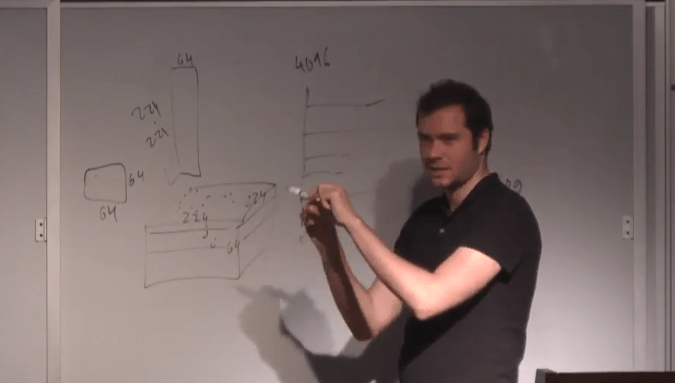Let’s use a CONV1 layer with 244 × 244 × 64 activations as an example:

We compute a 64 × 64 Gram matrix of all pairwise activation covariances summed across spatial locations.

To see this, we are converting a layer of activations ( which is a 3D 244 × 244 × 64 matrix) into a 2D matrix of size (244 × 244) × 64, and taking the outer product to form the 64 × 64 matrix. For each entry gi,j of the 64 × 64 matrix, we are multiplying together the activations of output channels i and j at that layer. If neurons in channels i and j fire together often across all spatial positions, they get added up and we will get a large element for gi,j .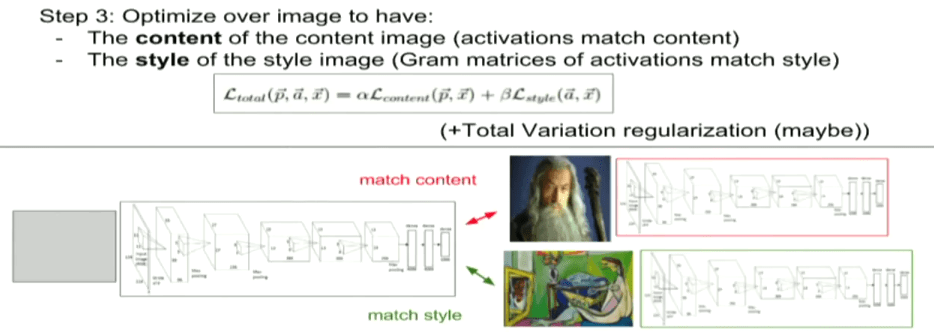So the Gram matrix G contains the statistics of which neurons fire together averaged across all spatial positions, and we compute a Gram matrix for each layer of the network. Finally, once we have these extractions, we optimize over image to have the content of Ic and the style of Is (see A Neural Algorithm of Artistic Style, Leon A. Gatys, Alexander S.Ecker, and Matthias Bethge, 2015 for more information).XI. Final Thoughts

In this review, I went over some of the techniques that can be used to understand and visualize neural networks. These techniques were gathered from many sources and were not presented in any order of importance.

We discussed how to visualize patches that maximally activate neurons, inspecting weights and their influences on the activations (section III). Visualizing the whole representation with techniques such as t-SNE (section IV). Occlusion experiements where we modified with in input and observe the output (section V). Several deconvolution approaches (section VI) followed by optimizing over the image (section VII) to maximize a class, firing rate of some neuron, or match a specific code. The idea of adversarial inputs for convolutional neural nets via a simplified linear explanation. Finally, we touched on some of the applications of optimizing over the image (final sections on deep dream, neural- style). These techniques reveal that the layers, or features of neural networks are not just random patterns, but have intuitive properties that can be understood. We can use these visualizations to identify problems with the model and obtain better results.

One final point of more practical value: the solution of today’s networks are proven empirically to be optimal as the neural networks grows, i.e. the gap between a “good” solution and a “bad” solution diminishes. Therefore, maybe being stuck in a local minima is no longer a concern?

Analyst: Joshua Chou | Localized by Synced Global Team : Xiang Chen